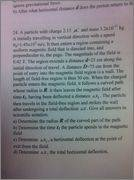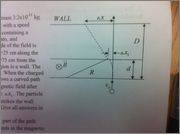# Tricky Magnetic Field Question (Proton Enters Magnetic Field )

Tricky Magnetic Field Question... (Proton Enters Magnetic Field......)

Here are 2 pictures.

1. This is the written question: (It's a long one.)2. Here is the given diagram for the question..Possible Equations to use:
fm = qvbsinA

Thoughts / attempts:

Initially i thought because the charge entered the field perpendicular to both the magnetic force.. that it would be a relation between Centrepetal force and magetnic force..

FM = FC

I solved for this and found the radius to be 5.18meters... which amkes no sense, so obviusly this technique can't be used..

Another strategy i'm thinking at the moment, is perhaps the angle at which the particle deviates could be related to the Radius and small (d) distance triangle... to solve using pythagereom thereom...

However i'm not sure how i could solve this angle, unless i relate the Magnetic Force to something.. Perhaps to Fnet = Ma, FM = Fnet

i'm also having a problem considering the accelleration, and i would like to know, Gravity is involved in the acceleration of this object at all, cause it does not mention that gravity is negligible,

Honestly, I just need a couple tips to be pointed in the right/ how to consider things... and last but not least...

I am really thinking, that the direction of the Force (FM) is in the direction where the charge is accelerating constantly(to the left), and that the direction it travels 'upward' from the diagram, is the direction in which the object travels in uniform motion, with no acceleration.. I believe this because the magnetic field vector is in the direction in which deflection occurs... (left) and thus must only be able to affect that 'horizontal' components.. leaving the vertical component unaffected...

Another reason i beleive there is no accelleartion 'vertically' (from diagram) is because the charges initially velocity, has only one component.. It reminds me of When you reach the Maximum H in a projectile... Where your component vertically is 0, and your horizontal component is (vx*T) in relation to this..

I can see that after entering the field, the Charge gets two velocity components...

So yea.. please give me some reccomendations ppzl <3

So yea any pointers... I don't wanna throw any solutions up yet.. I just want some advice before i put something half decent up and worth some ones time to look at.

I really want to treat it like a projectile... in some way shape or form *******

Last edited:

gneill
Mentor

Thoughts / attempts:

Initially i thought because the charge entered the field perpendicular to both the magnetic force.. that it would be a relation between Centrepetal force and magetnic force..

FM = FC

I solved for this and found the radius to be 5.18meters... which amkes no sense, so obviusly this technique can't be used..
Why do you think that this radius makes no sense? It's a radius of curvature of the path; it doesn't have to correspond to any particular dimension of the apparatus.
Another strategy i'm thinking at the moment, is perhaps the angle at which the particle deviates could be related to the Radius and small (d) distance triangle... to solve using pythagereom thereom...
Look at the diagram provided and where the shown radius vector is "rooted". The angle that this vector makes with the horizontal will be the same angle that the particle's path turns from the vertical (you should convince yourself that this is so).

At the outset you also might want to convince yourself that the effect of gravity will be negligible. What's the initial kinetic energy of the particle in the vertical direction, and what's the change in energy that can occur?

Why do you think that this radius makes no sense? It's a radius of curvature of the path; it doesn't have to correspond to any particular dimension of the apparatus.

Because.. I assumed that the radius would kind of moderately proportional to the diagram, which i should never think, but honestly i showed another student, and he's like that makes no sense... So i kind took his opinion.. which i shouldn't have...
Look at the diagram provided and where the shown radius vector is "rooted". The angle that this vector makes with the horizontal will be the same angle that the particle's path turns from the vertical (you should convince yourself that this is so).

Okay this is clear, thank you.
At the outset you also might want to convince yourself that the effect of gravity will be negligible. What's the initial kinetic energy of the particle in the vertical direction, and what's the change in energy that can occur?

Okay I'm going to start looking more closely at the energies, do you mind if i post back what i have in terms of a solution later tonight/tomorrow?

gneill
Mentor

Okay I'm going to start looking more closely at the energies, do you mind if i post back what i have in terms of a solution later tonight/tomorrow?

No problem. Take your timesince this is a uniform magnetic field, and the charge enters at a right angle,

the charge experiences circular motion. There is a constant force perpindicular to its motion.

therefore..
A)
Fm = Fc

R = mv/qb

R= 5.14m

b) now i was thinking in terms of that angle, if my original radius is correct, i should be able to make a trianglee... with the Radius R, and the distance (d) of the magnetic field

and solve..

SinA=d/R
A=2.79 degrees

now.. i don't really want to move on, yet because i'm not positive if this is an okay strategy to solve for this angle.

and in terms of energy, i did a calculation...

Et1= 1/2MVo^2 (initial point) potential energy lvl zero..

The change in energy i believe would be, no change.. There are no non conservative forces acting upon the charge...

and in circular motion work done on an object is = 0 because the force is perpindicular to the velocity..

so therefore the change in energy is 0...?

and this can be proven i noticed when i calculated

Et2 = 1/2Mvf^2 + mgd

If i make energy totals the same and solve for Vf

Et1 = Et1
1/2mvo^2 = mgd + 1/2mvf^2
Vf = sqrtall(mv^2 -2gd)
Vf = 145000
which is its original velocity

can you tell me how i'm doing :O

gneill
Mentor

since this is a uniform magnetic field, and the charge enters at a right angle,

the charge experiences circular motion. There is a constant force perpindicular to its motion.

therefore..
A)
Fm = Fc

R = mv/qb

R= 5.14m

b) now i was thinking in terms of that angle, if my original radius is correct, i should be able to make a trianglee... with the Radius R, and the distance (d) of the magnetic field

and solve..

SinA=d/R
A=2.79 degrees

now.. i don't really want to move on, yet because i'm not positive if this is an okay strategy to solve for this angle.
No, it works fine. That's a good value for the angle.
and in terms of energy, i did a calculation...

Et1= 1/2MVo^2 (initial point) potential energy lvl zero..

The change in energy i believe would be, no change.. There are no non conservative forces acting upon the charge...

and in circular motion work done on an object is = 0 because the force is perpindicular to the velocity..

so therefore the change in energy is 0...?

and this can be proven i noticed when i calculated

Et2 = 1/2Mvf^2 + mgd

If i make energy totals the same and solve for Vf

Et1 = Et1
1/2mvo^2 = mgd + 1/2mvf^2
Vf = sqrtall(mv^2 -2gd)
Vf = 145000
which is its original velocity

can you tell me how i'm doing :O
Doing okay so far.

For the check to make sure that gravity is an insignificant factor, you might have just compared the initial kinetic energy with the maximum change in potential energy across the extent of the apparatus.

but i have to solve from a time t1, and the x1 is the angle of deflection... i thought that kinematics would be required in that case ( b, and c)

Last edited:
gneill
Mentor

I don't understand why you're using formulas for motion under gravitational acceleration when you've decided that gravity can be ignored.

The particle's path curves while it is within the magnetic field, after which it travels in a straight line. After the "bend" in the trajectory due to the magnetic field, the problem boils down to geometry.

okay so..using the angle i had originally 2.79

i can use that angle to solve for x1
becaues the angle is the point which the proton deviates.

tanA = d/x1
x1=d/tanA

now in order to get t1 i need the hypoteneuse where it travels..

cosa = d/z
z= d/cosA

z = vot
t= z/vo